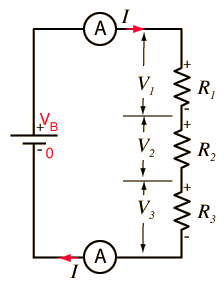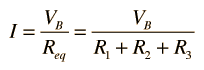# DC Series CircuitFor this series circuit, the voltage law dictates VB = V1 + V2 + V3 and the combination of resistors gives Requivalent = R1 + R2 + R3 In applying Ohm's Law with the battery voltage, you must realize that the battery voltage is applied to the total circuit (all three resistors) and not to any one of them alone. So the entire resistance must be used in Ohm's law to get the current:For R1 = Ω , R2 = Ω, R3 = Ω,

 the equivalent resistance is Requivalent = Ω.

 For battery voltage VB = V, the current is I = A.

Only after the current is determined is it convenient to calculate the individual voltages for the resistors. Applying Ohm's law again for each component gives

 V1 = V, V2 = V, V3 = V.

The values here should confirm the voltage law, i.e., that the sum of the component voltages must equal the battery voltage.

 DC circuit examples
Index

 HyperPhysics***** Electricity and Magnetism R Nave
Go Back